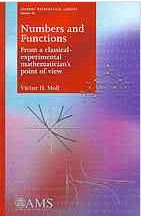## Numbers and Functions

### by Victor H. MollThe book covers an extensive swathe of college mathematics: number systems and number theory, special families of numbers (Fibonacci, Catalan, Stirling, Bernoulli), combinatorics, calculus and analysis, polynomials and special families of polynomials (Bernoulli, Chebyshev, Legendre, Hermite), Landen transformations, and special functions ($$\Gamma$$, $$\psi$$, $$\zeta$$). The book is by no means linear, with many references to later chapters which, I must say, does not at all hinder the reading but rather binds the material into coherent whole.

Every chapter contains an awful amount of surprising, non-routine mathematics. As I believe the author would put it, a few examples are given next.

In the first chapter, a brief outline of the axiomatics of natural numbers is followed by evaluating sums of powers $$\displaystyle\sum_{k}^{n}{k^n}$$. Among the methods employed are Gosper's automatic approach and Mathematica programming. Next come permutations, the multiplicative principle, and factorials, with a reference to N. Sloane's Online Encyclopedia of Integer Sequences. Divisibility and the Euclidean algorithm are immediately followed by Lamé's theorem on a bound for the length of the algorithm expressed by $$\mbox{log}_{10}b/\mbox{log}_{10}\phi$$, where $$b$$ is the smallest of the two numbers whose greatest common divisor is delivered by the algorithm and $$\phi$$ is the Golden Ratio. Then there are sections on modular arithmetic, with a demonstration of $$\displaystyle\sum_{j=1}^{n-1}\frac{1}{j}=\sum_{j=1}^{n-1}j\space(\mbox{mod}\space n)$$, for a prime $$n$$, implying that prime $$n$$ divides the numerator of the harmonic number $$H_{n-1}$$; next come prime factorization and $$p$$-adic valuation; the existence of infinite sets of pairwise coprime integers (and, hence, infinitude of primes); countability of rational numbers in a few ways, among which the Calkin-Wilf binary tree and D. Callan's "one-line" proof; continued fractions; irrationality of $$\sqrt{2}$$; real numbers and their rational approximation; Newton's method.

In the second chapter (Factorials and Binomial Coefficients), author proves various binomial congruences and Bertrand's postulate of the existence of prime $$p$$, $$n\le p\lt 2n$$, for $$n\gt 0$$; develops generating functions, in particular, for central binomial coefficients; proves Legendre's formula for the prime factorization of $$n!$$ and the Sterling formula.

Reluctantly - so as to keep this review to a manageable size - I skip to chapter 10 devoted to the Farey fractions and Stern-Brocot tree. Farey fractions have been extensively used to construct mathematical tables, the art of which appears to have long lost its significance with the advance of calculators and computers. It's surprising to learn that Mathematica's Rationalize[] command either does not use the Farey fractions, or has a bug, incorrectly giving $$\displaystyle\mbox{Rationalize}[Pi, 0.1]=\frac{22}{7}$$, instead of $$\displaystyle\frac{16}{5}$$. The chapter includes a discussion on the distribution of the denominators on the Stern-Brocot tree, and an algorithm for finding a fraction with the smallest denominator in a given interval.

Now I wish to address the second part of the book's title, From a classical-experimental mathematician's point of view. As the author writes in the Preface, Experimental mathematics is a relatively new name for an old approach to doing mathematics. Chapter 9 serves a good example. The task is to establish Wallis' Formula

$$\displaystyle W_{m}=\int_{0}^{\infty}\frac{dx}{(x^{2}+1)^{m+1}}$$, $$m\in\mathbb{N}_{0}.$$

The author starts with listing the first $$10$$ values of $$W_{m}$$ produced by Mathematics and draws the reader's attention to the fact that all $$10$$ are rational factors of $$\pi$$. He next observes that the denominators appear to be powers of $$2$$, with $$2$$-adic valuations given by

$$\displaystyle \{2,4,5,8,9,11,12,16,17,19\}.$$

This leads to a guess that the exponent is alway bounded by $$2m$$, which makes it reasonable to look for numbers

$$\displaystyle b_{m}=2^{2m}W_{m}/\pi.$$

These come out to be $$\{1,3,10,35,126,462,1716,6435,2431092378\}$$ and could be found at oeis.org. The search returns

$$\displaystyle b_{m}={2m-1 \choose m-1}=\frac{1}{2}{2m\choose m},$$

implying $$\displaystyle W_{m}=\frac{\pi}{2^{2m+1}}{2m\choose m}$$, which is to be proved. There is also another approach for guessing via factorization of $$b_m$$ using Mathematica. Then follow several proofs: a proof based on recurrences, a proof with generating functions, another proof based on recurrences but now applied to the trigonometric form of Wallis' formula; there also follows a related binomial sum identity and a section on an automatic proof that could be obtained with a single command entered in the WZ-package developed by H. Wilf and D. Zeilberger. Finally, there is a note to the effect that Wallis' formula is a consequence of a certain inequality that comes with references to its automatic derivation.

Chapter 9 reflects to a great extent on the author's approach throughout the book. What has to be proved (the classical part) is getting proved (often in multiple ways), the motivation for the result (or for a proof) is often procured using software and guessing (the classical-experimental part).

On the whole, this is an extraordinarily interesting book overflowing with (mostly) elementary mathematics. It's well written and a pleasure to read. I've been keeping it on my desk for the ease of access; it's going to stay there for some while. I recommend it wholeheartedly to math instructors, teachers and students, especially to those who have only a slight interest in the subject. The book is bound to expand their horizons.Numbers and Functions. From a classical-experimental mathematician's point of view, by Victor H. Moll. AMS, 2012. Softcover, 504 pp, \$58.00. ISBN 978-0821887950